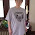## Wednesday, April 18, 2018

### How tall is the #OneWorldTradeTower? #OneWorldOrder #FreedomTower #1776

One World Trade Center (also known as 1 World Trade Center, 1 WTC or Freedom Tower.
40° 42′ 46.8″ N, 74° 0′ 48.6″ W

1,368 ft (417.0 m) to roof......

One World Order = 1368 (Jewish)
Key to the apocalypse" = 1368 (Jewish)
Haram esh-Sharif" = 417 (Jewish)

And while we're at it we will use this number for a couple of other things!

Gun control agenda. Parkland school  shooting 2/14/2018
February fourteenth" = 1368 (Jewish)

War with Russia agenda....

Russian Spy Poisoned" = 1368 (Jewish)
THANKS JEWS!

"September eleventh two thousand eighteen" = 417 (Jewish Ordinal)

Back to the New World Order.....

The reflection of 911 is 119

"Star of David" = 119 (English Ordinal)

"Tribe of Judah" = 119 (English Ordinal)
"All Seeing Eye" = 119 (English Ordinal)
"Foundation" = 119 (English Ordinal)
"Master Plan" = 119 (English Ordinal)
"Psychological operations" = 119 (Reverse  Full Reduction)
"sodomites" = 119 (English Ordinal)
"Suicide" = 119 (Reverse Ordinal)

Antenna is 408 ft tall
"United Airlines flight one seventy five" = 408 (English Ordinal)

Construction topped out on 5/10 2013
"United Airlines flight one seventy five" = 510 (Reverse Ordinal)

Architectural 1,776 ft (541.3 m)
"Federal Reserve System" = 1776 (Reverse English Sumerian)
"THIS NOTE IS LEGAL TENDER FOR ALL DEBTS PUBLIC AND PRIVATE" = 1776 (Francis Bacon)
The Order of the Skull and Bones" = 1776
(English Sumerian)
"star spangled banner" = 1776 (Reverse English Sumerian)
"February twenty seventh" = 1776 (English Sumerian)22/7=PI
"World Trade Buildings" = 1776 (Reverse English Sumerian)
"thirteenth of February" = 1776 (Reverse English Sumerian) 44th day of the year
"from sea to shining sea" = 1776 (Reverse English Sumerian)
"President George Bush" = 1776 (Reverse English Sumerian)
There are 1776 hours in 74 days
(Fourth of July is on 7/4)

Architectural 1,776 ft (541.3 m)

thirty three" = 541 (Satanic)
Twenty Eight" = 541 (Satanic)
left eye" = 541 (Jewish)
left eye" = 666 (Reverse English Sumerian)

"two eight five Fulton street" = 117 (Full Reduction)
"Central Intelligence Agency" = 117 (Full Reduction)

"Two hundred eighty five Fulton Street" = 156 (Septenary)911 is the 156th prime number

"Fulton Street" = 49 (Full Reduction)
square root 7..7*7=49
Twin Towers" = 49 (Full Reduction)
Anti Christ" = 49 (Full Reduction)
"Fulton Street" = 227 (Francis Bacon)

Dates.....

Construction started April 27, 2006
4+27+2+0+0+6=39
4+2+7+2+0+0+6=21
Construction stopped May 10, 2013
5+10+20+13=48
5+10+2+0+1+3=21
5+1+0+2+0+1+3=12
Topped-out May 10, 2013
Opened November 3, 2014
11+13+20+14=58
11+13+2+0+1+4=31
1+1+1+3+2+0+1+4=13
May 29, 2015 (One World Observatory)
5+29+20+15=69
5+29+2+0+1+5=42
5+2+9+2+0+1+5=24

Cost......
US\$3.9 billion
Master Mason" = 39 (Full Reduction)
Mark of Beast" = 39 (Full Reduction)

One world in Hebrew
33 (Hebrew Reduction)
"One World" = 106 (English Ordinal)
"Prophecy" = 106 (English Ordinal)

1.2.